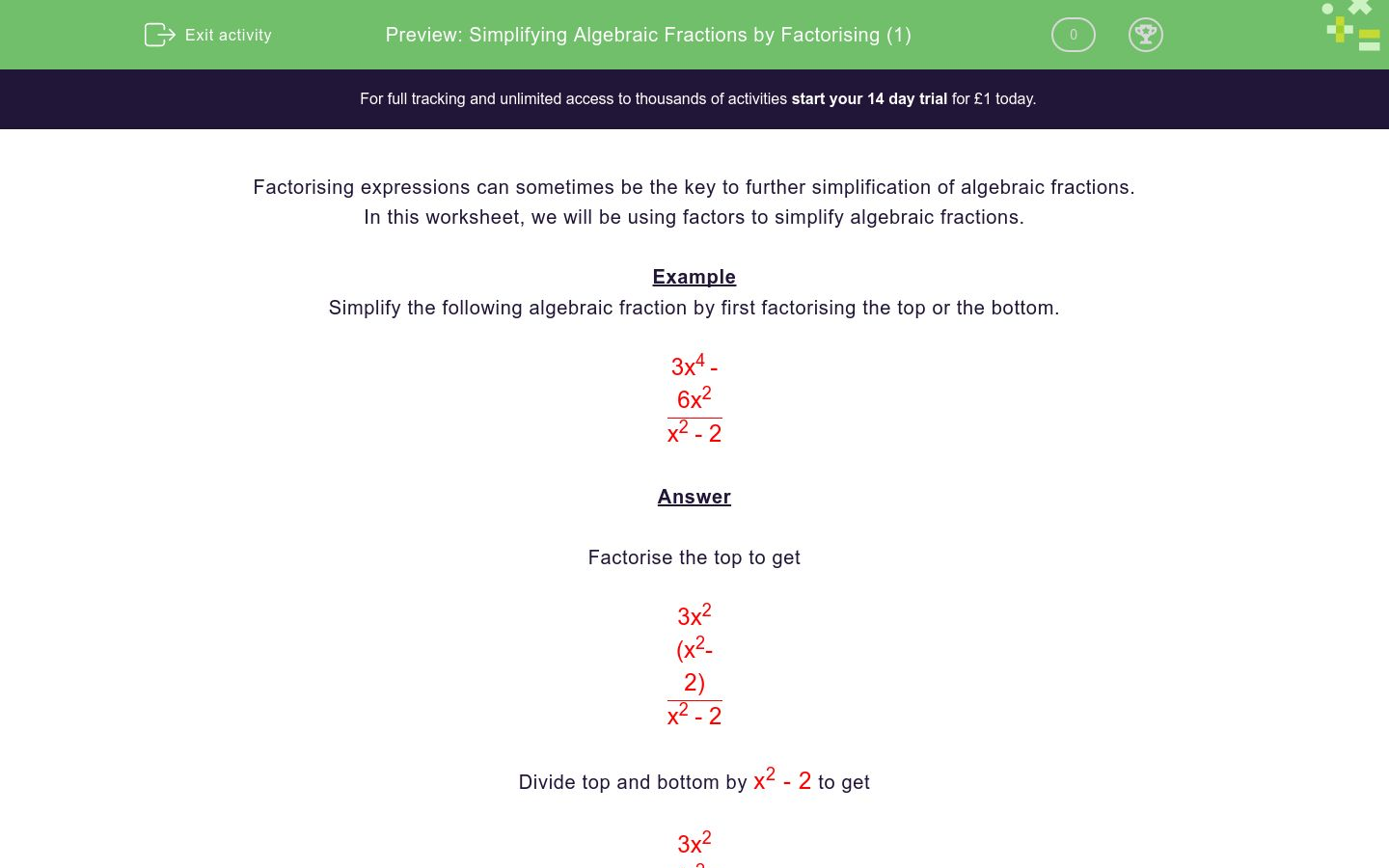# Simplifying Algebraic Fractions by Factorising (1)

In this worksheet, students simplify algebraic fractions by factorising.Key stage:  KS 4

Curriculum topic:  Algebra

Curriculum subtopic:  Simplify/Manipulate Algebraic Expressions/Fractions

Difficulty level:### QUESTION 1 of 10

Factorising expressions can sometimes be the key to further simplification of algebraic fractions.

In this worksheet, we will be using factors to simplify algebraic fractions.

Example

Simplify the following algebraic fraction by first factorising the top or the bottom.

 3x4 - 6x2 x2 - 2

Factorise the top to get

 3x2 (x2- 2) x2 - 2

Divide top and bottom by x2 - 2 to get

 3x2 (x2- 2) x2 - 2

3x2

Simplify the following algebraic fraction by first factorising the top or the bottom.

 3x2 - 5x 3x - 5

x

3x

3x - 5

Simplify the following algebraic fraction by first factorising the top or the bottom.

 9x2 - 15x 3x - 5

x

3x

3x - 5

Simplify the following algebraic fraction by first factorising the top or the bottom.

 6x2 + 9x 2x + 3

x

3x

3x - 5

Simplify the following algebraic fraction by first factorising the top or the bottom.

 2x2 - 9x 2x - 9

x

3x

3x - 5

Simplify the following algebraic fraction by first factorising the top or the bottom.

 25x2 - 15x 5x - 3

x

3x

5x

Simplify the following algebraic fraction by first factorising the top or the bottom.

 25x3 - 15x2 5x - 3

x

5x

5x²

Simplify the following algebraic fraction by first factorising the top or the bottom.

 18x3 - 18x2 3x - 3

6x²

6x

6x³

Simplify the following algebraic fraction by first factorising the top or the bottom.

 32x3 + 24x2 4x + 3

8x

8x²

8x³

Simplify the following algebraic fraction by first factorising the top or the bottom.

 32x4 + 24x3 4x + 3

8x

8x²

8x³

Simplify the following algebraic fraction by first factorising the top or the bottom.

 35x5 - 25x4 7x - 5

5x4

5x2

7x - 5

• Question 1

Simplify the following algebraic fraction by first factorising the top or the bottom.

 3x2 - 5x 3x - 5

x
EDDIE SAYS
 x(3x - 5) 3x - 5
• Question 2

Simplify the following algebraic fraction by first factorising the top or the bottom.

 9x2 - 15x 3x - 5

3x
EDDIE SAYS
 3x(3x - 5) 3x - 5
• Question 3

Simplify the following algebraic fraction by first factorising the top or the bottom.

 6x2 + 9x 2x + 3

3x
EDDIE SAYS
 3x(2x + 3) 2x + 3
• Question 4

Simplify the following algebraic fraction by first factorising the top or the bottom.

 2x2 - 9x 2x - 9

x
EDDIE SAYS
 x(2x - 9) 2x - 9
• Question 5

Simplify the following algebraic fraction by first factorising the top or the bottom.

 25x2 - 15x 5x - 3

5x
EDDIE SAYS
 5x(5x - 3) 5x - 3
• Question 6

Simplify the following algebraic fraction by first factorising the top or the bottom.

 25x3 - 15x2 5x - 3

5x²
EDDIE SAYS
 5x2(5x - 3) 5x - 3
• Question 7

Simplify the following algebraic fraction by first factorising the top or the bottom.

 18x3 - 18x2 3x - 3

6x²
EDDIE SAYS
 6x2(3x - 3) 3x - 3
• Question 8

Simplify the following algebraic fraction by first factorising the top or the bottom.

 32x3 + 24x2 4x + 3

8x²
EDDIE SAYS
 8x2(4x + 3) 4x + 3
• Question 9

Simplify the following algebraic fraction by first factorising the top or the bottom.

 32x4 + 24x3 4x + 3

8x³
EDDIE SAYS
 8x3(4x + 3) 4x + 3
• Question 10

Simplify the following algebraic fraction by first factorising the top or the bottom.

 35x5 - 25x4 7x - 5